Search IntMath
Close

450+ Math Lessons written by Math Professors and Teachers

5 Million+ Students Helped Each Year

1200+ Articles Written by Math Educators and Enthusiasts

Simplifying and Teaching Math for Over 23 Years

# IntMath Newsletter: Forum, pistons, Shodor

By Murray Bourne, 26 Jan 2016

26 Jan 2016

1. New: IntMath Forum
2. Piston curve
3. Resource: Shodor Interactivate
4. Math puzzles
5. Math movies: Misleading statistics
6. Final thought: Resolutions, 1% at a time

## 1. New: IntMath Forum

A lot of people have asked for a forum over the years. Finally, it's here!

IntMath Forum

I come across too many low quality forums and I'm hoping to avoid that. I also get a lot of mails containing math homework questions where the writer just wants me to provide the answer. This doesn't help him learn much - it just helps his marks. So there are 5 simple principles for the forum:

Principles of the IntMath Forum

You can enter math in the forum using simple plain text (or LaTeX if you prefer). You can also add graphs and images to your post. Details on how to do so can be found here:

Wanted: Volunteer Tutors

The forum needs tutors that embrace the 5 Principles mentioned above. That is, tutors should firstly be confident in answering the question, but help readers figure out problems by themselves, not just do it for them. The best help is given by prompting, reminding, and asking good questions - not simply providing the answer.

To get an idea what I mean, see these exchanges where I'm pointing the user to relevant resources, and asking questions. Eventually, they work it out:

Let me know (by replying to this mail, or in the comments) if you are interested to be a tutor.

## 2. Does a piston follow a sine curve shape?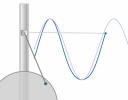In the Trigonometric Graphs section, I used a piston example for one of the sine curves. A reader pointed out that a piston does not exactly follow a sine curve at all. Here's a short article with an interactive animation that explores this issue: Curve Shape for the Movement of a Piston

## 3. Resource: Shodor's Interactivate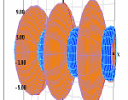I've been a fan of Shodor's Interactivate for many years. Indeed, it helped to inspire IntMath. Their activities used to be java-based, and so didn't work on mobile devices. But now, their interactive activities are mobile friendly. See over 300 activities, covering algebra, functions, ciphers, calculus, and probability here: Shodor's Interactivate

## 4. Math puzzles

The puzzle in the last IntMath Newsletter asked about the ratio of the areas of Reuleaux Triangles.

Correct answer with explanation was given by Stephen, who was also was one of the few to express the final answer as a ratio, as required.

### New math puzzle

The length of a rectangle increases by 10% and the width decreases by 10%. Which of the following is true?

• The area does not change.
• The area becomes smaller or larger depending on the original lengths of the sides.
• The area will always become smaller.
• The area will always become larger.

You can leave your responses here.

## 5. Math movie: Misleading statisticsThis video outlines Simpson's Paradox, which all budding statisticians need to be aware of. This is part of a TED-Ed lesson. How statistics can be misleading

### Related article: Misleading charts

Around the same time I watched How statistics can be misleading, I came across the following article on Quartz.

I feel it's a vital mathematical skill to be able to properly analyze data, especially when it's in visual form.

I don't agree with all the writer's suggestions, but overall it's quite a good analysis:

The most misleading charts of 2015, fixed

## 6. Final thought: Resolutions, 1% at a time

You probably made new year resolutions and now that life has taken over, maybe they've been shelved already.

A possibly more effective and realistic way of challenging yourself to improve is to aim for 1% improvement per day (or some other reasonably short time frame). If you want to exercise more, try just walking (or running, or any other exercise) 1% more than the day before. This will mean you'll double your walking distance in just 70 days:

(1.01)70 = 2.007

You could apply a similar small and realistic improvement goal for your studies: say 5% improvement for each weekly test.

Either way, all the best achieving your goals in 2016!

Until next time, enjoy whatever you learn.

See the 17 Comments below.

### 17 Comments on “IntMath Newsletter: Forum, pistons, Shodor”

1. Francis Kisner says:

Original Area = LW
New Area = (1.1 L)(.9 W) = .99 LW
The combination of the two changes always results in a decrease in the original area.

2. Rutwik Tank says:

Solution of the puzzle:
The area will always become smaller.
Explanation:
Let the length of the rectangle= x
and the width of the rectangle= y
Clearly, x>0, y>0
So the area of the rectangle= x*y
Now,
new length= x+(x/10)= (11*x)/10
new width= y-(y/10)= (9*y)/10
So the new area= (11x/10)*(9y/10)= 0.99*xy, which is always less than xy.

3. Kai says:

Rectangular Areas

Let the area of the original rectangle be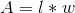If we increase the length by 10%, we have a new length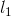given by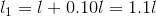Similarly, the new width will be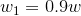The new rectangle then will have an area of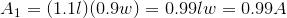So the new area will always be 99% of the old area - a 1% decrease

4. Dineth says:

Area will always become larger. (As length > width of a rectangle and area = length * width)

5. Jessica says:

Let L = original length and W = original width. Original area = L*W.
Length increases by 10%, so new length = 1.1*L
Width decreases by 10%, so new width = 0.9*W
New area = (1.1*L)(0.9*W) = 0.99*LW
Therefore, the new area is always 99% of the old area, so the new area is always smaller than the old area.

6. Don Miller says:

Always smaller

7. Tomas Garza says:

The area wll always become smaller. Call w the width and w + k the length (k > 0). Then the area is A = w (w + k). If the length is increased by 10% and the width decreased by 10%, the resulting area is 0.9 w times 1.1 (w + k) = 0.99 w (w + k) = 0.99 A, which is less than A.

8. Chloe says:

The areas will become :
Smaller or larger depending on the original values.

9. Andrzej Cichy says:

Consider a rectangle with the length a and width b.
Area before change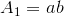The length a increases by 10% so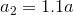The width b decreases by 10% so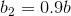Area after change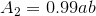, so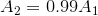So the area will always become smaller.

10. Nicos says:

Let the length be a and width b. Then the area E=a*b. If a is increased 10% and b decreased 10%, then a'=1.1*a and b'=0.9*b. The new area E'=a'*b'=1.1*a*0.9*b=0.99*a*b, so E'=0.99*E and the area will always become smaller, the 99% of the initial area.

11. michael says:

cute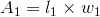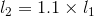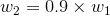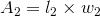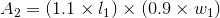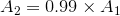The area will always become smaller.

12. Roger Evans says:

Hello,
The math puzzle...
The area will become smaller.
Take a rectangle 200 cms long with width 100 cms.
200 X +10% = 220 cms long, 100 X -10% = 90 cms width.
Multiply the original size out, subtract from the new size; the new rectangle has a smaller area.
Kind regards,
Roger

13. Guido says:

The area will always become smaller and exactly by 1% .
Area = L*B ----> 1.1L*0.9B = 0.99L*B

14. Nour Hlwani says:

Area of A rectangle =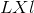Where L --> length and l --> width

L increased by 10% ->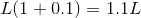l decreased by 10% ->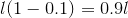The new area = 1.1×0.9×L×l = 0.99×(old area)
Since 0.99<1 Area is slightly decreasing

The right answer is (the area will alway become smaller.

15. Steve m. says:

The new area will always be smaller

i.e. new area= 0.99 * original area

16. Janet Teeguarden says:

The area will always get smaller, no matter which number is called length and which is width. New area = (L + 0.1L)(W - 0.1W) = LW + 0.1LW - 0.1LW - 0.01LW = LW - 0.01LW. So the new area is always one percent smaller than the original area LW. (This seems too easy...what did I miss here?)

17. KIPRONO ERIC says:

The area of the triangle will automatically reduce.the reduction will b an approximation of 1% as compared to the previous one.

### Comment Preview

HTML: You can use simple tags like <b>, <a href="...">, etc.

To enter math, you can can either:

1. Use simple calculator-like input in the following format (surround your math in backticks, or qq on tablet or phone):
a^2 = sqrt(b^2 + c^2)
(See more on ASCIIMath syntax); or
2. Use simple LaTeX in the following format. Surround your math with $$ and $$.
$$\int g dx = \sqrt{\frac{a}{b}}$$
(This is standard simple LaTeX.)

NOTE: You can mix both types of math entry in your comment.